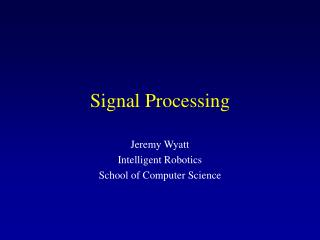DownloadDownload PresentationSignal Processing

# Signal Processing

Télécharger la présentation## Signal Processing

- - - - - - - - - - - - - - - - - - - - - - - - - - - E N D - - - - - - - - - - - - - - - - - - - - - - - - - - -
##### Presentation Transcript

1. Signal Processing Jeremy Wyatt Intelligent Robotics School of Computer Science

2. Sonar • Sound wave transmitter/receiver • Sound wave bounces back off objects • Counter measures time of flight • Calculates the distance assuming speed of sound is ~335ms-1 • Errors due to specular reflection

3. Metres per millisecond 0.5 msecs in each millisecond Sound travels twice the distance to the object Typical data • We gathered 100 samples from a sonar in three positions relative to a wall • You get a figure for time of flight (T) in units of 0.5 microseconds • To convert to metres

4. Median and mean • Order the values by size • take the central value, here 1.425 • mean is ~1.458 • median is more robust to outliers 1.41, 1.38, 1.71, 1.45, 1.42, 1.39, 1.43, 1.47 1.38, 1.39, 1.41, 1.42, 1.43, 1.45, 1.47, 1.71

5. Mapping • We took 25 sonar readings each at 100 equally spaced angles between 0 and 360 degrees

6. Plot range versus angle Range in metres Angle in degrees

7. Convert to Cartesian frame x y r q

8. (x,y) q r r

9. Finding Walls • Assume walls are straight lines • Each point is on many possible lines • Each line can be described by an equation

10. w f

11. Hough Transform Create f and w for all possible lines Create an array A indexed by f and w for each point (x,y) for each angle f w = x*cos(f)+ y*sin(f) A[f,w] = A[f,w]+1 end end where A > Threshold return a line

12. Summary • Use median • Hough finds lines • But because of width of sonar beam gives bad results • May be better with Infra Red • Smooth in Hough Space • Use property of sonar